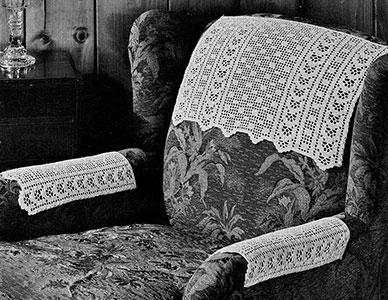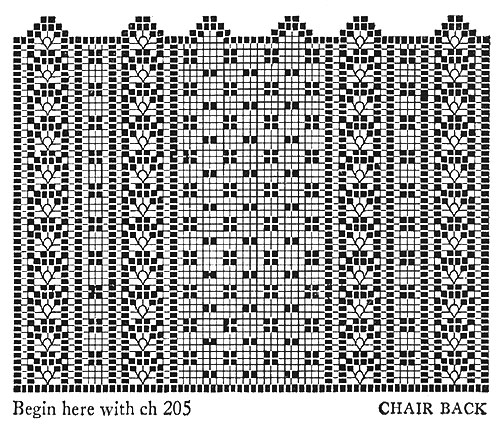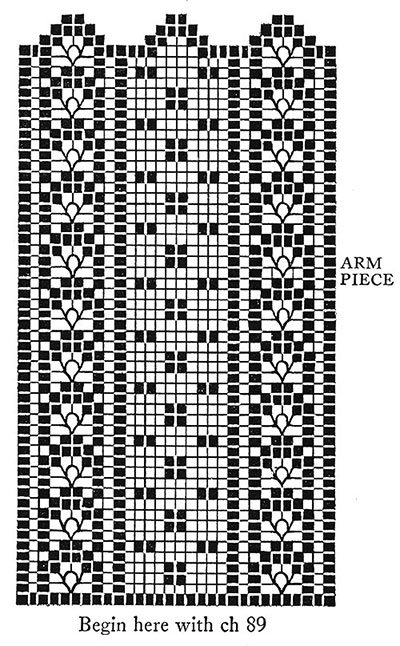## Checker Filet Chair Set Pattern #7038Printer-friendly versionMaterials: J. & P. Coats Knit-Cro-Sheen, 3 balls of color 61 Ecru. Milward's steel crochet hook No. 4.

When completed, chair back measures about 19½ x 13 inches and each arm piece about 8 x 13 inches.

Chair Back. Ch 205, turn. 1st row: D c in 4th ch from hook, 1 d c in each st of foundation ch (203 d c counting turning ch as 1 d c). Ch 3, turn. 2nd row: * D c in each of next 3 d c, ch 2, skip 2 d c, d c in each of next 4 d c, ch 5, skip 4 d c, s c in next d c, ch 5, skip 4 d c, d c in each of next 4 d c, ch 2, skip 2 d c, d c in each of next 4 d c, ch 2, skip 2 d c, d c in next d c (1 sp), make 3 more sps. Then make d c in each of next 3 d c, ch 2, skip 2 d c, d c in each of next 4 d c, ch 5, skip 4 d c, s c in next d c, ch 5, skip 4 d c, d c in each of next 4 d c, ch 2, skip 2 d c, d c in each of next 4 d c, ch 2, skip 2 d c, d c in next d c (1 sp), make 21 more sps. Then repeat from * ending row with d c in each of last 4 d c. Ch 3, turn. 3rd row: D c in each of the next 3 d c, ch 2, d c in each of next 4 d c, ch 5, s c in first loop, ch 5, s c in next loop, ch 5, d c in each of next 4 d c, ch 2, d c in each of next 4 d c, ch 2, d c in next d c, ch 2, d c in next d c, ch 2, d c in next d c, ch 2, d c in each of next 4 d c, ch 2, d c in each of next 4 d c, ch 5, s c in first loop, ch 5, s c in next loop, ch 5, d c in each of next 4 d c, ch 2, d c in each of next 4 d c. * Make sp over each of next 4 sps of previous row. 2 d c in next sp, d c in next d c, 2 d c in next sp, d c in next d c. Repeat from * twice more. Then make sp over each of next 4 sps of previous row, d c in each of next 3 d c, ch 2 and complete row to correspond with beginning, ending row with d c in each of the last 4 d c. Ch 3, turn. 4th row: D c in each of next 3 d c, ch 2, d c in each of next 4 d c, 3 d c in first loop, ch 2, d c in next loop, ch 2, 3 d c in next loop, d c in each of next 4 d c, ch 2, d c in each of next 4 d c. Make sp over each of next 4 sps, d c in each of next 3 d c, ch 2, d c in each of next 4 d c, 3 d c in first loop, ch 2, d c in next loop, ch 2, 3 d c in next loop, d c in each of next 4 d c, ch 2, d c in each of next 4 d c. Make 22 sps, d c in each of next 3 d c, ch 2, and complete row to correspond with beginning, ending row with d c in each of the last 4 d c. Ch 3, turn. 5th row: D c in each of next 3 d c, ch 2, d c in first d c of group, ch 2, skip 2 d c, d c in each of next 4 d c, 2 d c in sp, d c in next d c, 2 d c in next sp, d c in each of next 4 d c, ch 2, skip 2 d c, d c in next d c, ch 2, d c in each of next 4 d c, ch 2, d c in next d c, 2 d c in sp, d c in next d c, 2 d c in next sp, d c in next d c, ch 2, d c in each of next 4 d c, ch 2, d c in first d c of group, ch 2, skip 2 d c, d c in each of next 4 d c, 2 d c in sp, d c in next d c, 2 d c in next sp, d c in each of next 4 d c, ch 2, skip 2 d c, d c in next d c, ch 2, d c in each of next 4 d c, ch 2, d c in next d c, 2 d c in sp, d c in next d c, 2 d c in next sp, d c in next d c. Make sp over each of next 4 sps and complete row following chart. Hereafter follow chart until 53 rows are made. Then ch 1, turn.

To Make Scallops: 1st row: Sl st over 6 d c, ch 3, d c in each of the next 3 d c, 3 d c in sp, ch 2, d c in next sp, ch 2, 3 d c in next sp, d c in each of next 4 d c, turn.

2nd row: Sl st over 3 d c, ch 3, d c in each of next 3 d c, 2 d c in sp, d c in d c, 2 d c in next sp, d c in each of next 4 d c, turn. 3rd row: Sl st over 3 dc, ch 3, d c in each of next 6 d c. Fasten and break off. A scallop is made over the 4 sps. Make scallops over each group of 4 sps across the top in the same way.

Arm Piece. 1st row: Ch 89, turn. 2nd row: D c in 4th ch from hook, and d c in each st of foundation ch (87 d c). Ch 3, turn. Hereafter follow chart and make scallops in the same way as for chair back. Make another arm piece same as this one.